## 前言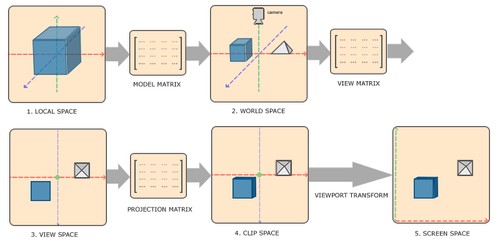## 坐标系变换矩阵

\begin{pmatrix} U_{xx} & U_{yx} & U_{zx} & O_{x}\\ U_{xy} & U_{yy} & U_{zy} & O_{y}\\ U_{xz} & U_{yz} & U_{zz} & O_{z}\\ 0 & 0 & 0 & 1 \end{pmatrix}^{-1} \\[2em] \begin{aligned} \overrightarrow{U_{x}} &= (U_{xx},\ U_{xy},\ U_{xz}),\ \text{x轴基向量} \\ \overrightarrow{U_{y}} &= (U_{yx},\ U_{yy},\ U_{yz}),\ \text{y轴基向量} \\ \overrightarrow{U_{z}} &= (U_{zx},\ U_{zy},\ U_{zz}),\ \text{z轴基向量} \\ \overrightarrow{O} &= (O_{x},\ O_{y},\ O_{z}),\ \text{原点} \end{aligned}

：左乘是因为得到的变换矩阵是列主序的，为啥矩阵要列主序？因为glsl中的矩阵就是列主序的

## 模型变换矩阵

M = T * R * S \\[1em] \begin{aligned} T&: \text{平移矩阵} \\ R&: \text{旋转矩阵} \\ S&: \text{缩放矩阵} \end{aligned}

1. 平移后会影响旋转，因此这里的旋转指的是是绕模型中心进行的旋转操作；所以平移要后于旋转进行。
2. 同样，旋转后再进行缩放时，可能会造成缩放的效果不对（主要是旋转后x, y, z坐标发生改变，而缩放x, y, z系数不一致时，就可能对应不上）；因此缩放要先于旋转进行。

## 视图变换矩阵

### 相机空间

• VRP（View Reference Point）：观察参考点，也叫视点（eyepoint）；相机坐标系的原点。
• VPN（View Plane Normal）：观察平面法向量，也叫观察/相机方向；相机坐标系的z轴。
• VUV（View Up Vector）：相机顶部正朝向；相机坐标系的y轴大概方向。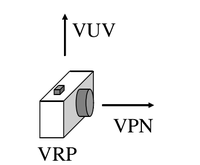### 推导过程

1. 首先，相机坐标系原点（即人眼或相机位置）在世界坐标系中的位置是已知的；相机的关注点（lookat）的位置也是已知的；VUV也是已知的；
2. 根据关注点和相机位置可以得到VPN（即相机坐标系的z轴基向量，$\overrightarrow{N}$）；
3. 根据VUVVPN叉乘可以得到相机坐标系的x轴基向量，$\overrightarrow{U}$
4. 同理，根据$\overrightarrow{N}$$\overrightarrow{U}$的叉乘可以得到真正y轴基向量，$\overrightarrow{V}$
5. $\overrightarrow{U}$$\overrightarrow{V}$$\overrightarrow{N}$以及VRP的坐标代入到通用变换公式即可得到视图变换矩阵$V$

\begin{aligned} V &= \begin{pmatrix} u_{x} & v_{x} & n_{x} & O_{x}\\ u_{y} & v_{y} & n_{y} & O_{y}\\ u_{z} & v_{z} & n_{z} & O_{z}\\ 0 & 0 & 0 & 1 \end{pmatrix}^{-1} \\[2em] &= (T * R)^{-1} \\ &= R^{-1} * T^{-1} \\ &= \begin{pmatrix} u_{x} & u_{y} & u_{z} & -\overrightarrow{U}\cdot O\\ v_{x} & v_{y} & v_{z} & -\overrightarrow{V}\cdot O\\ n_{x} & n_{y} & n_{z} & -\overrightarrow{N}\cdot O\\ 0 & 0 & 0 & 1 \end{pmatrix} \end{aligned} \\[2em] \begin{aligned} R &= \begin{pmatrix} u_{x} & v_{x} & n_{x} & 0\\ u_{y} & v_{y} & n_{y} & 0\\ u_{z} & v_{z} & n_{z} & 0\\ 0 & 0 & 0 & 1 \end{pmatrix} \\[2em] T &= \begin{pmatrix} 1 & 0 & 0 & O_{x}\\ 0 & 1 & 0 & O_{y}\\ 0 & 0 & 1 & O_{z}\\ 0 & 0 & 0 & 1 \end{pmatrix} \\[2em] R^{-1} = R^T &= \begin{pmatrix} u_{x} & u_{y} & u_{z} & 0\\ v_{x} & v_{y} & v_{z} & 0\\ n_{x} & n_{y} & n_{z} & 0\\ 0 & 0 & 0 & 1 \end{pmatrix} \\[2em] T^{-1} &= \begin{pmatrix} 1 & 0 & 0 & -O_{x}\\ 0 & 1 & 0 & -O_{y}\\ 0 & 0 & 1 & -O_{z}\\ 0 & 0 & 0 & 1 \end{pmatrix} \end{aligned}

：当一个矩阵为正交矩阵时（即行向量和列向量两两正交），其转置矩阵等于其逆矩阵

## 投影变换矩阵

### 相关概念

1. 投影点：相机坐标系的原点。
2. 近平面：通过设置近平面距离$D_{near}$可得，所以近平面指的是相机坐标系$z = -D_{near}$平面。
3. 远平面：同理，设置一个远平面距离$D_{far}$可得，近平面指的是相机坐标系$z = -D_{far}$平面。
4. 平截头体的宽高：指的是平截头体在近平面一端上的矩形宽高（远平面一端矩形的宽高可以根据参数推算）。

### 正交投影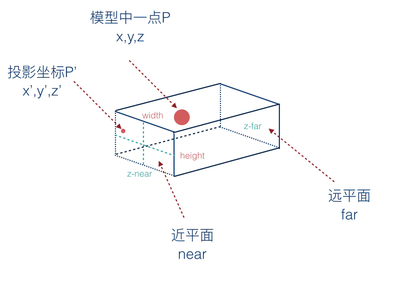：正交投影平截头体的宽高一般是通过设置$x_{left}$$x_{right}$$y_{top}$$y_{bottom}$得到的。

\begin{aligned} x' &= \frac{x - x_{left}}{x_{right} - x_{left}} * 2 - 1 \\ &= \frac{2 * (x - x_{left}) - x_{right} + x_{left}}{x_{right} - x_{left}} \\ &= \frac{2x - (x_{left} + x_{right})}{x_{right} - x_{left}} \\ &= \frac{2x}{x_{right} - x_{left}} - \frac{x_{left} + x_{right}}{x_{right} - x_{left}} \end{aligned}

\begin{aligned} y' &= \frac{2y}{y_{top} - y_{bottom}} - \frac{y_{bottom} + y_{top}}{y_{top} - y_{bottom}} \\ z' &= \frac{2z}{z_{near} - z_{far}} - \frac{z_{far} + z_{near}}{z_{near} - z_{far}} \end{aligned}

：由于相机坐标系是右手系，因此$z_{near} > z_{far}$

$P_{正交} = \begin{pmatrix} \large \frac{2}{\large x_{right} - x_{left}} & 0 & 0 & - \large \frac{\large x_{left} + x_{right}}{\large x_{right} - x_{left}} \\[1em] 0 & \large \frac{2}{\large y_{top} - y_{bottom}} & 0 & - \large \frac{\large y_{bottom} + y_{top}}{\large y_{top} - y_{bottom}} \\[1em] 0 & 0 & \large \frac{2}{\large z_{near} - z_{far}} & -\large \frac{\large z_{far} + z_{near}}{\large z_{near} - z_{far}} \\[1em] 0 & 0 & 0 & 1 \end{pmatrix}$

### 透视投影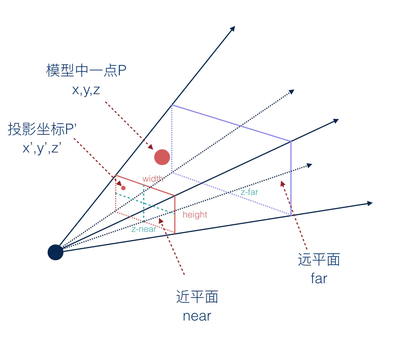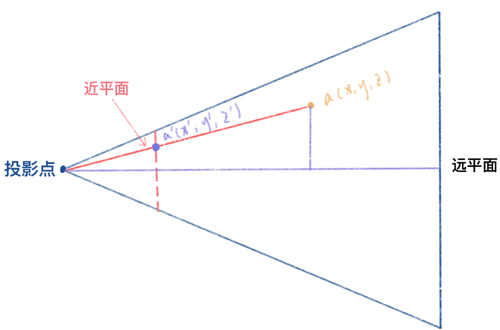$\frac{x'}{x} = \frac{y'}{y} = \frac{z_{near}}{z} \\[1em] \Rightarrow \large\begin{cases} x' &= \frac{z_{near} * x}{z} \\[1em] y' &= \frac{z_{near} * y}{z} \\[1em] z' &= z_{near} \end{cases}$

\begin{aligned} x'' &= \frac{x' - x_{left}}{x_{right} - x_{left}} * 2 - 1 \\ &= \frac{x' - (-width / 2)}{width} * 2 - 1 \\ &= \frac{2x'}{width} \\[2em] &= \frac{z_{near} * 2x}{z * width} \\[2em] y''&= \frac{z_{near} * 2y}{z * height} \end{aligned}

\begin{aligned} x'' &= - \frac{z_{near} * 2x}{width} \\[2em] y''&= - \frac{z_{near} * 2y}{height} \end{aligned}

$z'' = a\cdot\frac{1}{\large z} + b$

$\begin{cases} a * \large\frac{1}{\large z_{near}} + b &= -1 \\[1em] a * \large\frac{1}{\large z_{far}} + b &= 1 \end{cases} \\[1em] \Rightarrow \begin{cases} a &= \large \frac{\large 2 * z_{near} * z_{far}}{\large z_{near} - z_{far}} \\[1em] b &= - \large \frac{\large {\large z_{near} + z_{far}}}{\large z_{near} - z_{far}} \end{cases} \\$

$P_{透视} = \begin{pmatrix} \large \frac{2 * \large z_{near}}{width} & 0 & 0 & 0 \\[1em] 0 & \large \frac{2 * \large z_{near}}{height} & 0 & 0 \\[1em] 0 & 0 & \large \frac{\large {\large z_{near} + z_{far}}}{\large z_{near} - z_{far}} & \large \frac{2 * \large z_{near} * z_{far}}{\large z_{near} - z_{far}} \\[1em] 0 & 0 & -1 & 0 \end{pmatrix}$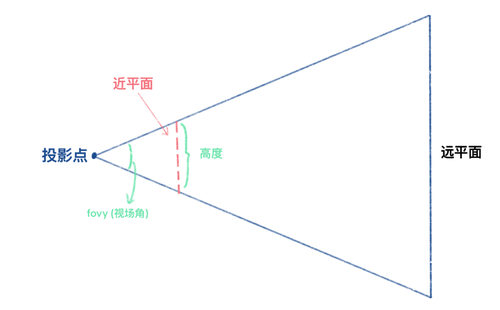$\begin{cases} tan(fovy / 2) &= \large \frac{\large 0.5 * height}{\large z_{near}} \\[1em] aspect &= \large \frac{\large width}{\large height} \end{cases} \\[1em] \text{记 }\theta = fovy / 2 \\[1em] \Rightarrow P_{透视} = \begin{pmatrix} \large \frac{cot(\theta)}{aspect} & 0 & 0 & 0 \\[1em] 0 & cot(\theta) & 0 & 0 \\[1em] 0 & 0 & \large \frac{\large {\large z_{near} + z_{far}}}{\large z_{near} - z_{far}} & \large \frac{2 * \large z_{near} * z_{far}}{\large z_{near} - z_{far}} \\[1em] 0 & 0 & -1 & 0 \end{pmatrix}$